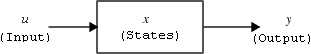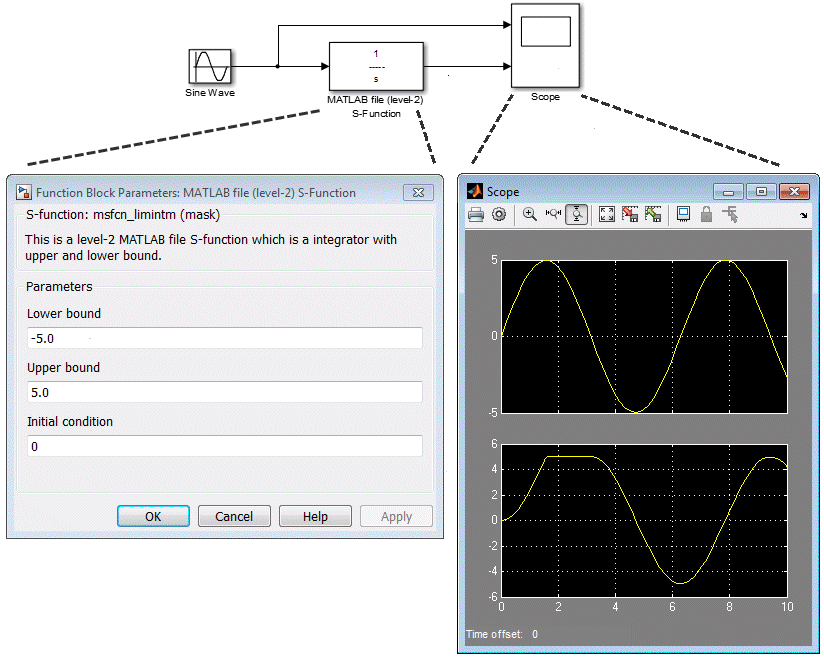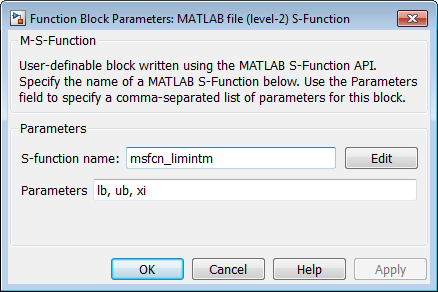## What Is an S-Function?

S-functions (system-functions) provide a powerful mechanism for extending the capabilities of the Simulink® environment. An S-function is a computer language description of a Simulink block written in MATLAB®, C, C++, or Fortran. C, C++, and Fortran S-functions are compiled as MEX files using the `mex` utility (see Build C MEX Function). As with other MEX files, S-functions are dynamically linked subroutines that the MATLAB execution engine can automatically load and execute.

S-functions use a special calling syntax called the S-function API that enables you to interact with the Simulink engine. This interaction is very similar to the interaction that takes place between the engine and built-in Simulink blocks.

S-functions follow a general form and can accommodate continuous, discrete, and hybrid systems. By following a set of simple rules, you can implement an algorithm in an S-function and use the S-Function block to add it to a Simulink model. After you write your S-function and place its name in an S-Function block (available in the User-Defined Functions block library), you can customize the user interface using masking (see Create Block Masks).

If you have Simulink Coder™, you can use S-functions in a model and generate code. You can also customize the code generated for S-functions by writing a Target Language Compiler (TLC) file. For more information, see S-Functions and Code Generation (Simulink Coder).

### How S-Functions Work

S-functions define how a block works during different parts of simulation, such as initialization, update, derivatives, outputs and termination. In every step of a simulation, a method is invoked by the simulation engine to fulfill a specific task. S-function basics require fundamental knowledge of mathematical relationships between the block inputs, states, and outputs. To understand how S-functions work, first you need to understand the mathematics of how Simulink simulates a model, namely the stages of simulation. See Simulation Phases in Dynamic Systems for more detailed information.

#### Mathematics of Simulink Blocks

A Simulink block consists of a set of inputs, a set of states, a set of parameters, and a set of outputs, where the outputs are a function of the simulation time, the inputs, parameters, and the states.The following equations express the mathematical relationships between the inputs, outputs, parameters, states, and simulation time.

where $x=\left[{x}_{c};{x}_{d}\right].$

#### Simulation Stages

Execution of a Simulink model proceeds in stages. In the Initialization phase, the Simulink engine incorporates library blocks into the model, propagates signal widths, data types, and sample times, evaluates block parameters, determines block execution order, and allocates memory. The engine then enters a simulation loop, where each pass through the loop is referred to as a simulation step. During each simulation step, the engine executes each block in the model in the order determined during initialization. For each block, the engine invokes functions that compute the block states, derivatives, and outputs for the current sample time. The entire simulation loop then continues until the simulation is complete.

Model Initialization - Model is prepared for simulation. In this stage, block parameters are evaluated, block execution order is determined, and memory for each operation is allocated. After this stage, blocks go through a simulation loop.

Update of Continuous States and Time - Takes place only if the model has continuous states. You can modify minor step methods such as `mdlOutputs`, `mdlDerivatives`, and `mdlZeroCrossing` to compute the outputs

#### S-Function Callback Methods

An S-function comprises a set of S-function callback methods that perform tasks required at each simulation stage. During simulation of a model, at each simulation stage, the Simulink engine calls the appropriate methods for each S-Function block in the model. Tasks performed by S-function callback methods include:

• Compilation — In this stage, the Simulink engine initializes the S-function. Tasks include:

• Incorporating library blocks into the model, and propagating signal widths, data types, and sample times

• Setting the number and dimensions of input and output ports

• Evaluating block parameters, and determining the block execution order

• Allocating memory and storage areas.

• Calculation of outputs — At this state, outputs are calculated until all the block output ports are valid for the current time step, namely all output values are at a certain error range.

• Update discrete states — In this call, the block performs once-per-time-step activities such as updating discrete states.

• Initialize and Terminate Methods — These optional methods perform initialization and termination activities required by S-function only once. The initialization activities may include setting up user data, or initializing state vectors in an S-function. The termination method performs any actions such as freeing of memory that is required when the simulation is terminated, or when an S-function block is deleted from a model.

• Integration — This applies to models with continuous states and/or nonsampled zero crossings. If your S-function has continuous states, the engine calls the output and derivative portions of your S-function at minor time steps. This is so the solvers can compute the states for your S-function. If your S-function has nonsampled zero crossings, the engine also calls the output and zero-crossings portions of your S-function at minor time steps so that it can locate the zero crossings.

To understand the terminology on simulations especially for S-functions, see S-Function Concepts.

### Use S-Functions in Models

1. To incorporate a C MEX S-function in a model, drag a S-function block from the Simulink Library Browser. Similarly, to incorporate a Level-2 MATLAB S-function to the model, drag a Level-2 MATLAB S-function block to the model.

2. Open up the Block Parameters dialog and specify the S-function name at the S-function name field to provide functionality for the S-function block. For example, type `timestwo` and hit to add a C MEX S-function that multiplies the incoming signal by two.

Note

If the MATLAB path includes a C MEX file and a MATLAB file having the same name referenced by an S-Function block, the S-Function block uses the C MEX file.

#### Passing Parameters to S-Functions

In both S-function block and Level-2 MATLAB S-Function Block Parameters window allows you to specify parameter values to pass to the corresponding S-function. To use these fields, you must know the parameters the S-function requires and the order in which the function requires them. (If you do not know, consult the S-function's author, documentation, or source code.) Enter the parameters, separated by a comma, in the order required by the S-function. The parameter values can be constants, names of variables defined in the MATLAB or model workspace, or MATLAB expressions.

The following example illustrates usage of the Parameters field to enter user-defined parameters for a Level-2 MATLAB S-function.The model `msfcndemo_limintm` in this example incorporates the sample S-function `msfcn_limintm.m`.The `msfcn_limintm.m` S-function accepts three parameters: a lower bound, an upper bound, and an initial condition. The S-function outputs the time integral of the input signal if the time integral is between the lower and upper bounds, the lower bound if the time integral is less than the lower bound, and the upper bound if the time integral is greater than the upper bound. The dialog box in the example specifies a lower and upper bound and an initial condition of `-5.0`, `5.0`, and `0`, respectively. The scope shows the resulting output when the input is a sine wave of amplitude 5.

See Processing S-Function Parameters and Handle Errors in S-Functions for information on how to access user-specified parameters in an S-function.

You can use the masking facility to create custom dialog boxes and icons for your S-Function blocks. Masked dialog boxes can make it easier to specify additional parameters for S-functions. For a discussion on masking, see Create Block Masks.

#### When to Use an S-Function

You can use S-functions for a variety of applications, including:

The most common use of S-functions is to create custom Simulink blocks (see Block Creation Basics). When you use an S-function to create a general-purpose block, you can use it many times in a model, varying parameters with each instance of the block.